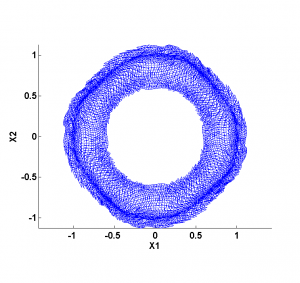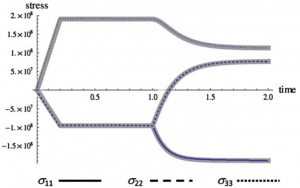# if-then

Assertions of truth can usually be phrased in the form “if … then …”. Such assertions can be re-stated in many other equivalent ways. Doing so helps you to better understand these fundamental truths or to easily expose falsehoods.

Below are two infographics summarizing the main ways to re-phrase if-then statements. Click here for a PDF document that gives details (especially explaining what is meant by the word “implies”). The PDF also offers additional examples and in-class exercises.

This example is trivial to help make it clear that truth of one implies truth of all others (and untruth of one would also imply untruth of all others). But these sorts of rephrasings can give you unexpected insights when applied to nontrivial if-them statements. Try it on a few of the examples in the full PDF document!

# Particle basis function in the CPDI method

The Convected Particle Domain Interpolation (CPDI) method for evaluating nodal integrals in the weak form of the momentum equation employs a dynamically adaptive alternative to standard shape functions on the grid. The CPDI basis functions change depending on the underlying particle morphology.  The animations in this post each provide image triplet groupings, one triplet for pure translation and the other for uniform stretching, defined as follows:

• TOP IMAGE: The dynamic CPDI basis (solid lines) compared with the conventional FEM-style basis functions (shaded tent functions). The adaptive CPDI basis is constructed to exactly coincide with the conventional FEM basis at particle edges (called corners), and it varies linearly across each particle domain, thus making integrals of the basis or its gradient over a particle domain quite simple. The CPDI basis is linearly complete.
• MIDDLE IMAGE: The particle basis (shown shaded in dark gray). Like the CPDI basis itself, the particle basis is never constructed explicitly. We are visualizing it here to better understand the domain of influence of a particle and (we hope) to discourage the mysterious tendency for researchers to refer to$\phi_i(x_p)=\frac{1}{V_p}\int_{\Omega_p(x_p)}S_i(x)dx$ as the particle basis function.  To the contrary, and as explained in detail in the bottom of this post, the particle basis function is the coefficient of the particle data value appearing in an expansion of a field as it is used in the discretization of governing equations.  The solution of the governing equations uses a mapping of particle data to the grid. Consequently, because all fields are treated as expansions on the grid, the particle basis function (found by setting the particle value to 1 and all other particle data to zero) must be a grid expansion (so it MUST be piecewise linear on the grid if using linear shape functions).  This proper definition of the particle basis function is shown below in dark gray. The particle basis function is NOT the top hat function (shown in light gray), nor is it$\phi_{ip}$ as commonly and misleadingly asserted. The relatively complicated derivation of the particle basis function is provided at the bottom of this post.  In the images below, two options are considered for the mapping$u_p$ particle data to the grid nodal value$u_i=\sum_p\psi_{pi}^*u_p$:
• Option 1:$\psi_{pi}^*=\frac{\phi_{ip}^*}{\sum_p\phi_{ip}}$
• Option 2:$\psi_{pi}^*$ is taken as the pseudo-inverse of$\phi_{ip}^*$

Here,$\phi_{ip}^*$ is the average of the ith CPDI grid nodal basis function,$S_i^*(x)$ over the pth particle domain$\Omega_p$. The GIMP variants of MPM  are similar except that they evaluate$\phi_{ip}$ and$\psi_{pi}$ using the conventional$S_i(x)$ grid basis functions rather than the adaptive (and actually computationally simpler) ones used in CPDI. Legacy “standard” MPM evaluates$\phi_{ip}$ to merely equal$S_i(x_p)$, which causes grid-crossing errors. The figure groupings below use the CPDI formula$\phi_{ip}=\frac{1}{V_p}\int_{\Omega_p}S_i^*(x) dx$ in which$S_i^*(x)$ is the CPDI adaptive grid basis. The MIDDLE image in each image triplet shows the particle basis, which is constructed by applying the above mapping to the grid with all particle values set to 0 except 1 at the particle of interest. The light grey filled box in the middle image shows a piecewise constant description (=1 on the particle domain and 0 elsewhere), while the dark filled function is the associated particle basis on the grid. The dots show nodal values of the particle basis function to emphasize that nodal values are not equal to field values (there is no need for them to be). Seeing a nodal value “pop up” gives you a sense of when a particle begins to influence the field in grid cells containing that node. The other (colored) graphs in that plot are the similarly constructed basis functions for the other particles. As seen in the upcoming plots, OPTION 2 (the pseudo-inverse) produces particle basis functions that are neither positive everywhere nor of compact support (which could be problematic for parallelisation in applications).

• The BOTTOM row in each grouping of plots shows the representations of a field that is constant (at particles) and a field that linearly varying from 0 to 1 over the physical domain (with particle values set equal to that field at the particle location). As seen, OPTION 2 for setting$\psi_{pi}^*$ gives excellent results for the linear field when there are at least two particles per cell, but OPTION 1 seems to be a better overall choice because it gives good results for any particle density, including fractional particles per cell (desired for coarse descriptions in regions of little interest). Grid errors at boundaries with OPTION 1 could probably be reduced by enriching boundary particles (as described in the CPDI2 publication), but this remains to be proved.

# Publication: Establishing credibility of particle methods through verification testingEvidence of discretization texture bias in a simulation that is supposed to exhibit polar symmetry

ABSTRACT:

﻿Within the particle methods community, standard benchmark tests are needed to demonstrate that the governing equations are solved correctly.Whereas the finite element method (FEM) has long-established basic verification standards (patch tests, convergence testing, etc.), no such standards have been universally adopted within the particle method community. Continue reading

# Validation of the Plasticity Modes and Introduction of Hardening Laws

These are power-point slides that I used during my lectures in the Computational Constitutive Modelling on October 25 and 27, 2011. The power-point slides can be found here.

# Elastic Constants Converter

This program allows one to convert between the various elastic constants.  If “Evaluate” is chosen in the downloadable version, the numbers entered below the chosen elastic properties are calculated, otherwise the Mathematica file displays the results symbolically. Continue reading

# Primer on von Mises (J2) plasticityBelow is a link to a primer showing how to write a very simple von Mises plasticity model using the classical radial return method.  Highlighted in yellow you will see an important warning about the limitation of such models. Nevertheless, this primer is a good place for a beginner to start. Also, the last two pages of this primer describe two very simple verification tests, which anyone who runs a plasticity model in a code should always test first.

Tutorial On J2 Plasticity

# Delft Short Course: excerpts of discussion of basis and frame indifference

This posting links to a pdf,  DelftExcerpts, which contains slides taken from a 2004 short course given in TU Delft (Netherlands) on the mathematics of tensor analysis.  Following a review of the mathematics of line integrals, inexact differentials, and integrability, this set of slides provides some insight into the distinction between a global basis change (equivalent to the “space rotation” in the slides) and superimposed rotation.  It also provides an introduction to the principle of material frame indifference (PMFI) as it applies to restricting allowable forms and input/output variables of computational constitutive models.